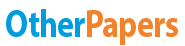Reflection of Light - a Lab Report

Essay by   •  April 13, 2018  •  Lab Report  •  407 Words (2 Pages)  •  1,385 Views

Essay Preview: Reflection of Light - a Lab Report

Report this essay
Page 1 of 2

Reflection of Light

[pic 1]

Analysis:

1. How far behind the mirror is the image of an object that is located in front of the mirror?

The distance of the image behind the mirror is the same distance as the object in front of the mirror, so the distance is the same.

2. Compare the size of your original object triangle with the size of your constructed image of the triangle.

When comparing the size of the original triangle with the size of the constructed image of the triangle, the object of the triangle retains the same size as the image of the triangle.

3. A ray of light is incident upon a mirror at an angle of 30˚. What is the angle between the incident ray and the reflected ray?

If a ray of light were to have an incident angle of 30˚,the incident ray will have an angle of 60˚ , as the angle of incidence is equal to 90˚ - 30˚, which is 60˚. The angle of reflection is equal to the angle of incidence, therefore the angle of incidence is 60˚ as well. The angle between the incident and reflected ray will be 60˚ + 60˚ = 120˚

4. If A is the left vertex of your original triangle, is the vertex A’ of the image of the triangle the left or the right vertex?

If A is the left vertex of my triangle, Vertex A’ of the image of the triangle will be the right vertex, as the image is reversed from left to right.

5. When a candle is placed between two vertical mirrors forming a right angle, three images of the candle are seen. Make a diagram showing their positions. If the angle between the mirrors is decreased to 60˚, how any images are now formed? Make a diagram showing their positions.

On sheet

Conclusion:

In conducting this lab, we observed how a plane mirror would reflect the pin. We aligned a ruler to the image of the object in the mirror. The image was reversed from left to right, displaying a property of mirrors. We were able to determine the ray of reflection and noticed that the angle of incidence was different from the angle of reflection, which was all due to human error. The lab allowed us to examine the law of reflection in the demonstration with the plane mirror, furthering our background information regarding how an object follows this law.

...

...